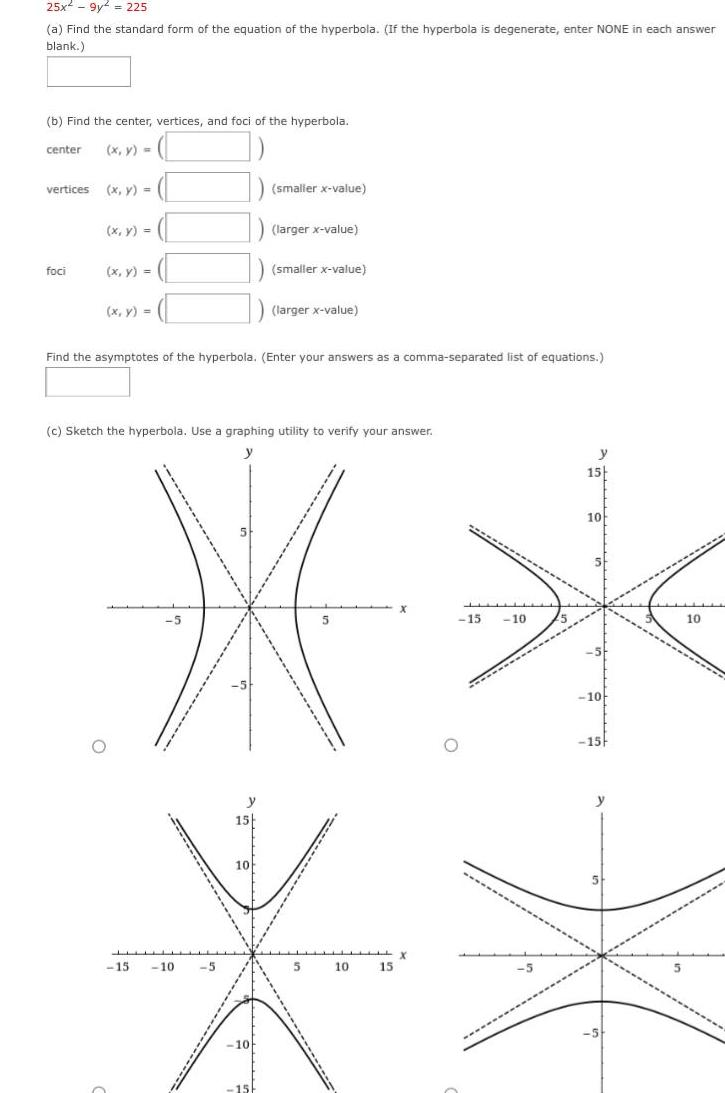Question:

# 25x 9y 225 a Find the standard form of the equation of the

Last updated: 5/11/202325x 9y 225 a Find the standard form of the equation of the hyperbola If the hyperbola is degenerate enter NONE in each answer blank b Find the center vertices and foci of the hyperbola center x y vertices x y 1 x y foci x y x y 15 10 5 15 Find the asymptotes of the hyperbola Enter your answers as a comma separated list of equations 10 smaller x value c Sketch the hyperbola Use a graphing utility to verify your answer y 5 15 10 5 10 15 10 larger x value 151 smaller x value larger x value y 15 10 5 10 15 y 5 10